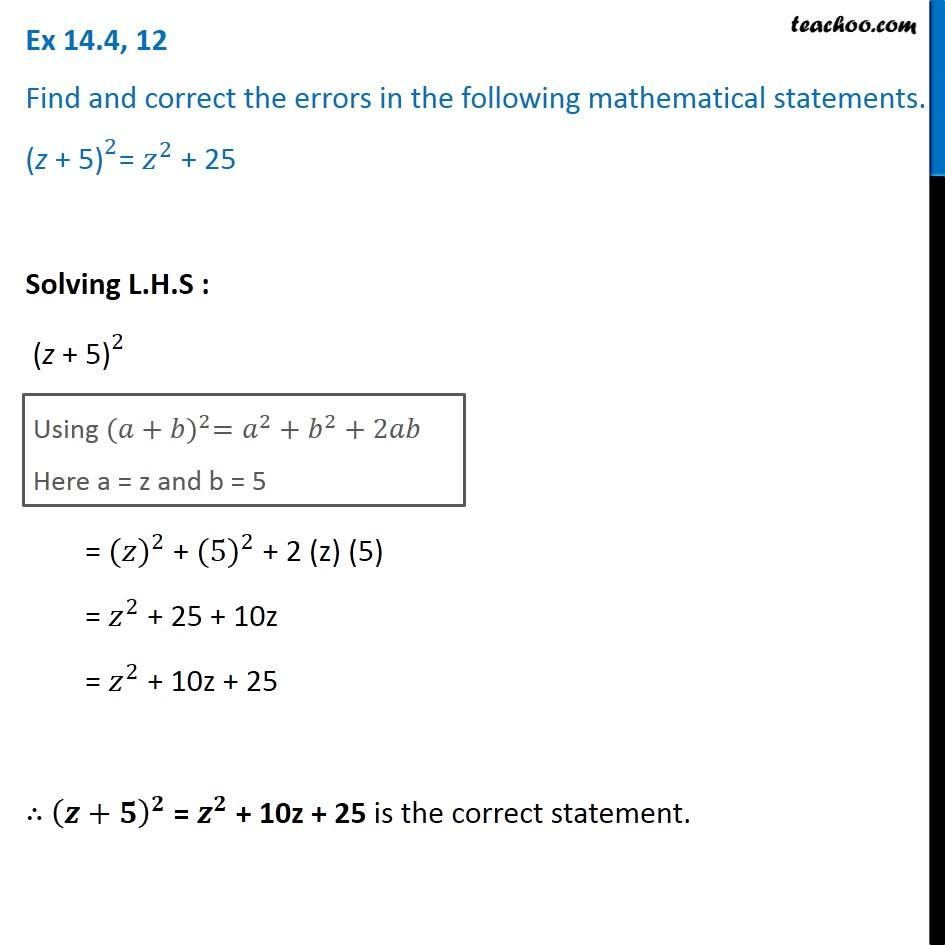Identify and correct errors in the sentences

Chapter 12 Class 8 Factorisation
Serial order wiseLearn in your speed, with individual attention - Teachoo Maths 1-on-1 Class

### Transcript

Question 12 Find and correct the errors in the following mathematical statements. 〖"(z + 5)" 〗^2= 𝑧^2 + 25 Solving L.H.S : 〖"(z + 5)" 〗^2 Using 〖(𝑎+𝑏)〗^2=𝑎^2+𝑏^2+2𝑎𝑏 Here a = z and b = 5 = 〖(𝑧)〗^2 + 〖(5)〗^2 + 2 (z) (5) = 𝑧^2 + 25 + 10z = 𝑧^2 + 10z + 25 ∴ (𝒛+𝟓)^𝟐 = 𝒛^𝟐 + 10z + 25 is the correct statement.